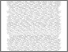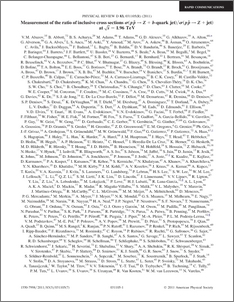# A measurement of the ratio of inclusive cross sections $σ(p\bar{p}\rightarrow Z+b{\rm\, jet})/ σ(p\bar{p}\rightarrow Z+{\rm jet})$ at $\sqrt{s}=1.96$ TeV

Collaboration, D0 and Bertram, Iain and Borissov, Guennadi and Fox, Harald and Williams, Mark and Ratoff, Peter and Love, Peter and Sopczak, Andre (2011) A measurement of the ratio of inclusive cross sections $σ(p\bar{p}\rightarrow Z+b{\rm\, jet})/ σ(p\bar{p}\rightarrow Z+{\rm jet})$ at $\sqrt{s}=1.96$ TeV. Physical Review D, 83 (3). ISSN 1550-7998Preview
PDF
PhysRevD.83.031105.pdf - Published Version

## Abstract

The ratio of the cross section for $p\bar{p}$ interactions producing a $Z$ boson and at least one $b$ quark jet to the inclusive $Z+{\rm jet}$ cross section is measured using $4.2\ {\rm fb}^{-1}$ of $p\bar{p}$ collisions collected with the \dzero\ detector at the Fermilab Tevatron collider at $\sqrt{s}=1.96$ TeV. The $Z\rightarrow\ell^+\ell^-$ candidate events with at least one $b$ jet are discriminated from $Z+$ charm and light jet(s) events by a novel technique that exploits the properties of the tracks associated to the jet. The measured ratio is $0.0193\pm0.0027$ for events having a jet with transverse momentum $\pt > 20 \GeV$ and pseudorapidity $|\eta| \leq 2.5$, which is the most precise to date and is consistent with theoretical predictions.

Item Type:
Journal Article
Journal or Publication Title:
Physical Review D